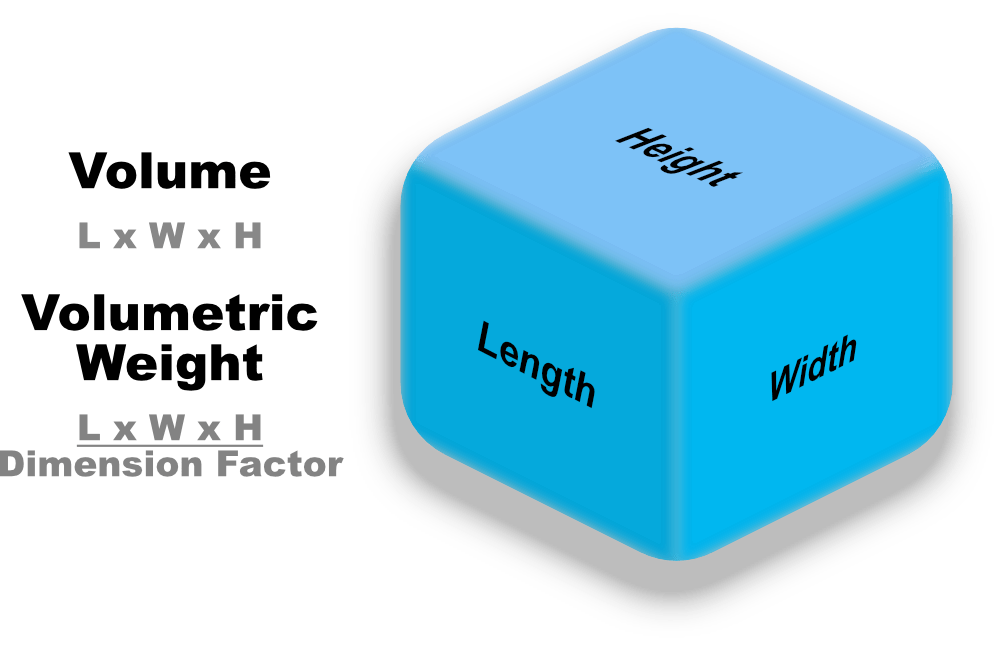# What Is Volumetric Weight and How Is It Calculated?

### Written by Andrew Lee

Volumetric weight is a way to calculate the volume of a package, rather than the weight itself. This is especially true with lightweight items that take up a certain amount of space. Why not stick with either weight or volume? To answer that question, read below.

This concept might make more sense if the roles were reversed.  Let’s play devil’s advocate for a moment, where you are the shipper. To further simplify this idea, let’s make all shipping costs equal, no matter which method (air, land, or sea). Knowing that there’s a numerical limitation in weight and space on any given solution; land, air, or sea. Which is more important, the weight or the volume? In truth, this depends on how you, the shipping carrier, decide to charge. This is called the chargeable weight. Many of the major shipping carriers charge depending on whichever weight is greater. If the package dimension weight is greater, they will charge by dimensional weight, and if the weight is greater, they will charge by the actual weight. Whichever is greater, will be the billable weight.

For example, let’s say that there are two sellers, one who sells pillows, and the other selling mobile phones. Would you charge the pillow vendor the same amount as you would charge the cell phone vendor? The phones use less space (volume), but weigh more. In contrast, the pillows have less weight but much more volume. To think of it another way, one pound of cotton vs 1 pound of iron. You might think that the one pound iron would cost more, but in logistical terms, the cotton is more costly. Why? It’s because the name of the game is optimization. Calculating the most effective way to optimize the space available in whichever shipping methods the client chooses.

## What is Volumetric Weight?

Normally, the freight carrier will charge by weight, but with anything, there are exceptions to the rule. Case in point, lightweight item(s) compared to their size (volume). To avoid any confusion, the industry standardized a minimum weight based on shipment dimensions. This calculation applies to packages larger than 1 Cubic Foot or 1728 Cubic Inches.

## Why does volumetric weight matter?

Volumetric weight, also known as dimensional weight, DIM weight, volumetric weight, volume weight or cubed weight, is a pricing technique that allows shipping carriers to calculate the true shipping rates for either the greater weight, either in actual weight or volume based weight.

## What is Actual Weight?

As the term sounds, actual weight is when only the weight of the package is calculated in the cost structure. This occurs when the volumetric weight is less than the actual weight. While, the dimensional weight will calculate the volume of the package into the equation, which is then converted into its equivalent weight.

## How is Volumetric or Dimensional Weight Calculated?

What You’ll Need:

1. Package Measurements (L x W x H)
2. Determine the dimensional factor. This is the number you will divide the volume by. This number will change depending on which mode of transportation you choose (air, sea, land).

## How to Calculate the Volumetric Weight

Volumetric weight is calculated by multiplying Volume / volumetric factor. Where volume is calculated by multiplying the length x height x width of a package, and the volumetric factor or DIM factor is calculated directly by the shipping carrier. PNPLINE, a premier logistics provider, has their own proprietary dim factor. Where we can help you save even more in your shipping strategy.## Should Volumetric Weight Be Divided By 5000?

There are numerous variables involved in this practice, but it is most commonly used for international shipments. Note that there are many other factors to look at. For instance, the mode of transportation, is it a domestic or international shipment, is it inland or delivered to another island? At the end of the day, the charges will be determined by the true weight (gross weight) or the volumetric weight, whichever weight is greater. Note that sometimes shipping carriers use 4000 m3/kg.

## What is the chargeable weight?

In simple terms, the chargeable weight is either the actual weight (gross weight), or the volumetric weight. This will be calculated, compared, and charged accordingly to the client. With additional costs depending on the packaging and services rendered.

## How to Calculate Chargeable Weight for Air Freight?

Akin to the base criteria of volumetric weight or the actual weight, air freight chargeable weight is determined by which weight is greater. Where dimensional weight is calculated by finding the volume (Length x Width x Height). However, the DIM factor will be different.

1. To convert inches into kilograms, one will have to divide the cubic inch by 366 (366 cu in/kg)
2. To convert centimeters into kilograms, one will have to divide. the cubic centimeter by 6000 (6000 ccm/kg)
3. To convert inches to pounds, one will have to divide the cubic inch by 166 cubic inches per pound (166 cu in/lb)

## How to Calculate the Chargeable Weight for Sea Freight?

Since cargo ships can handle significantly more weight and volume than air or land transportation methods, the calculation will be different. Due to the dim factor being low (around one ton or 1,000 kg per cubic meter) in the volumetric weight calculation, LCL (Less than Container Load) costs are almost all calculated with the volumetric weight. Where a pallet is the minimum size.

## Summary

Now that you know what volumetric weight is, and how it is calculated, if you need further assistance, PNPLINE will help you with your shipping strategy to a more affordable freight forwarding experience.